Scatter Plot
• 14 Mar 2023
• Dark
Light

# Scatter Plot

• Dark
Light

The Scatter Plot excels at showcasing change over time, especially for continuous values. Here are some examples: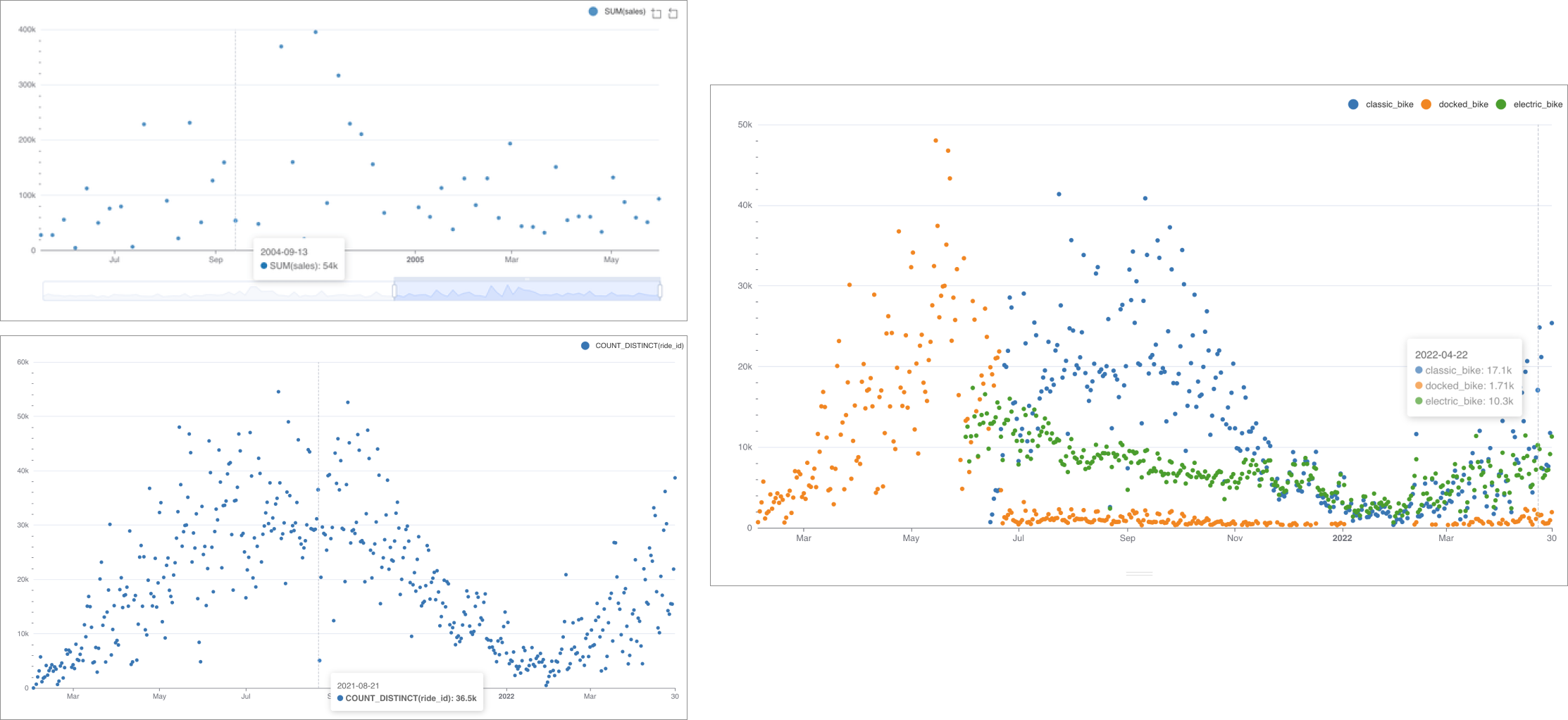### Reference Content

If you're new to visualizing data in Preset, we recommend the following reference articles:

## Creating a Scatter Plot

To create a Scatter Plot, you need to define the following values:

• the column you want as the X-axis
• the metric(s) you want to visualize on the Y-axis
• the column(s) you want the metric(s) to be grouped / categorized by

These are all defined in the Data tab within Chart Builder.

Simple Scatter Plot (no dimensions)

Below is a very simple scatter plot that shows a single metric varying over time.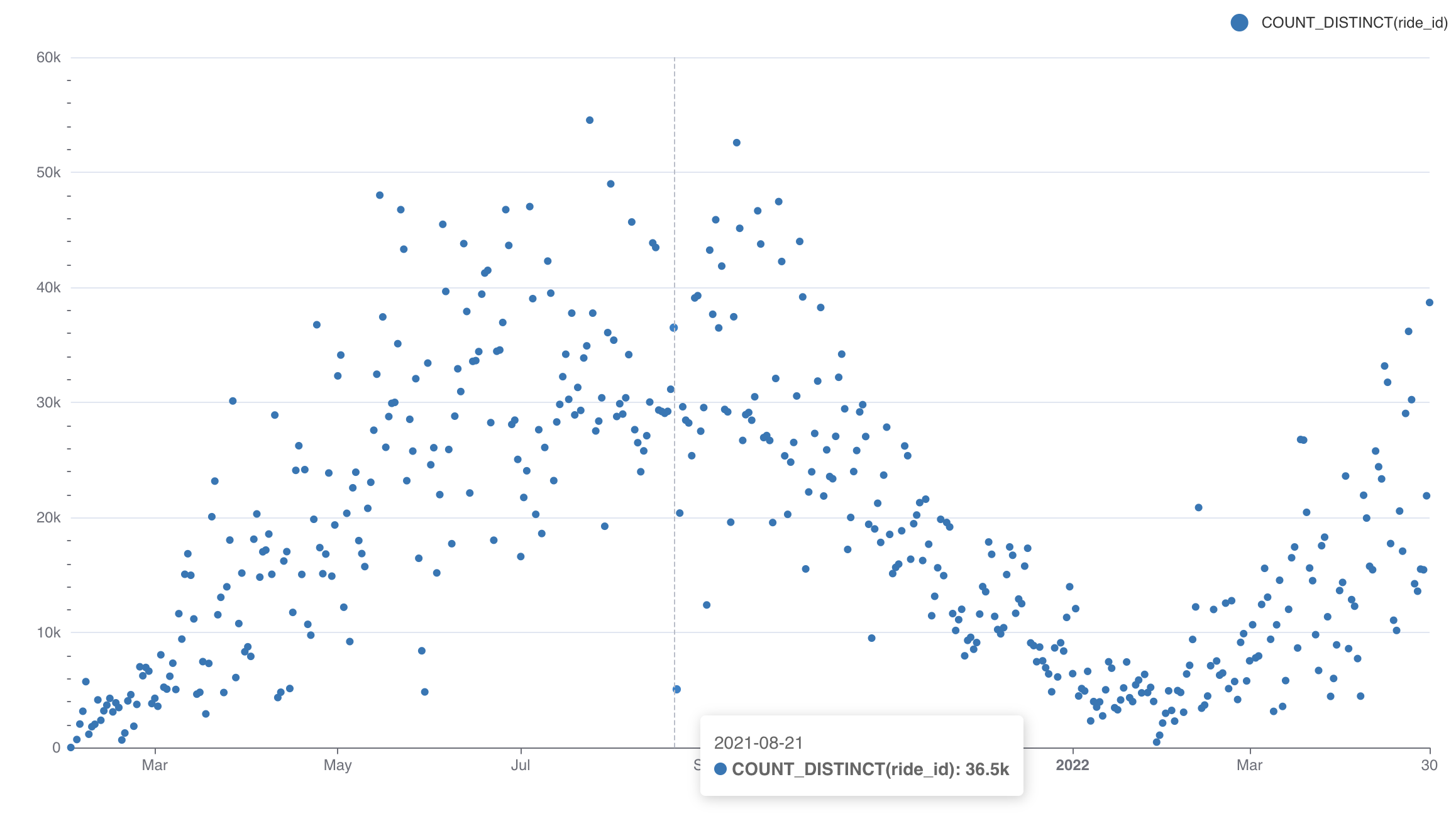Here's an explanation of the selections we made in the chart builder interface to generate this chart.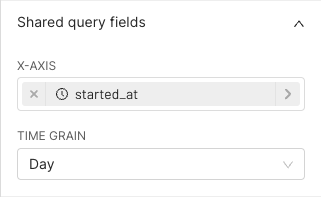X-axisX-axis: Select the column (e.g., Time, Categories) to be visualized.Time Grain: Define the granularity of the X-axis (Hourly, Daily, Weekly, etc.). The field will appear for temporal dimensions only.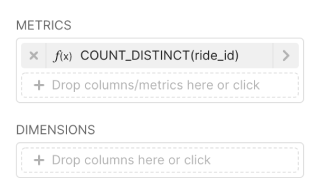Metric(s) on Y-axisDrag or select the Metric(s) to be visualized on the Y-axis.

Here's the SQL query that Preset generated:

``````SELECT DATE_TRUNC('DAY', started_at) AS "started_at",
count(DISTINCT ride_id) AS "COUNT_DISTINCT(ride_id)"
FROM dbt_smukherjee.citibike_trips
WHERE started_at >= '2022-04-01 00:00:00.000000'
AND started_at < '2022-05-01 00:00:00.000000'
GROUP BY DATE_TRUNC('DAY', started_at)
ORDER BY "COUNT_DISTINCT(ride_id)" DESC
LIMIT 10000``````

This hopefully helps you understand the mapping from the chart builder options above to the final generated SQL query.

Scatter Plot (with dimensions)

Below is a similar chart to the one above but with a dimension (bike type) added.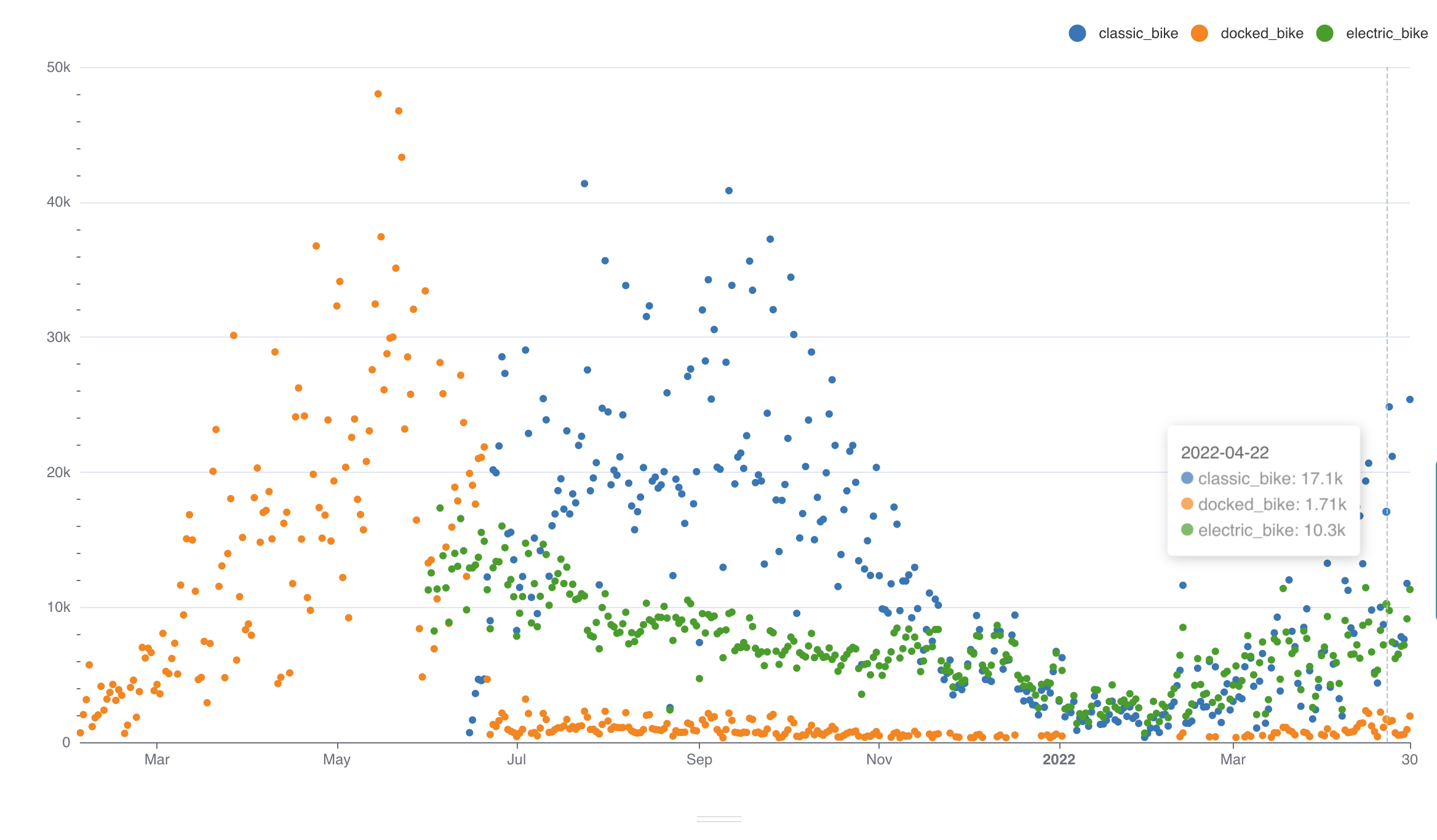To generate this chart, we re-used the same selections from the first chart but made the following, additional selections: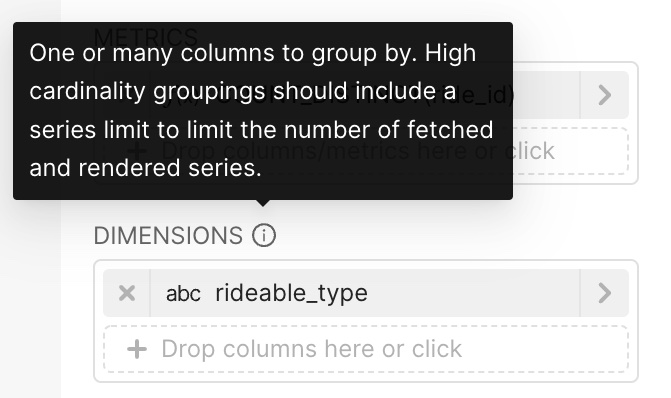DimensionsSelect any columns you want to slice/separate the series objects by. The unique values for those columns will be used to group together values and generate each X series object.

Here's the SQL query that Preset generated:

``````SELECT DATE_TRUNC('DAY', started_at) AS "started_at",
rideable_type AS "rideable_type",
count(DISTINCT ride_id) AS "COUNT_DISTINCT(ride_id)"
FROM dbt_smukherjee.non_member_trips
GROUP BY DATE_TRUNC('DAY', started_at),
rideable_type
ORDER BY "COUNT_DISTINCT(ride_id)" DESC``````